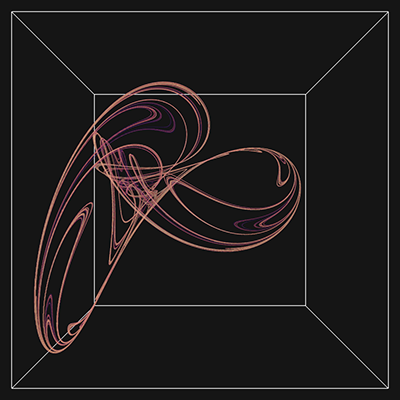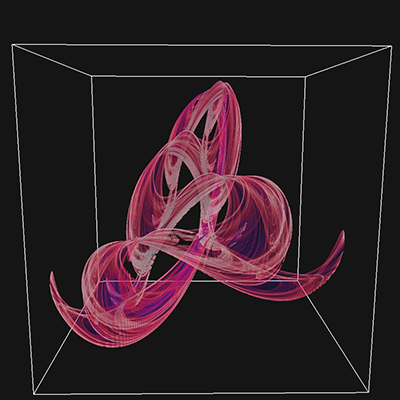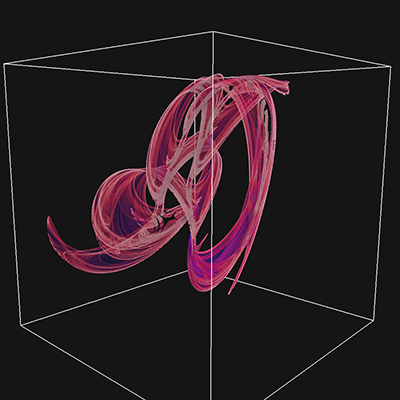# Polynomial attractor

Written by Paul Bourke
September 2020

I rediscovered the original article by J.C.Sprott (Sprott, J. C. "Automatic Generation of Strange Attractors." Comput. & Graphics 17, 325-332, 1993c) that was one of the inspirations for my fractal explorations. The images in that publication are rather simplistic, not a complaint but a fact of graphics in that era. I didn't readily find other example and the ones on Wolfram Mathworld were equally uninspiring. So I decided to produce some myself using my 2D histogram method along with a collection of colour maps.

The attractors are based upon the equations below.

xn+1 = a0 + a1xn + a2xn2 + a3xnyn + a4yn + a5yn2
yn+1 = a6 + a7xn + a8xn2 + a9xnyn + a10yn + a11yn2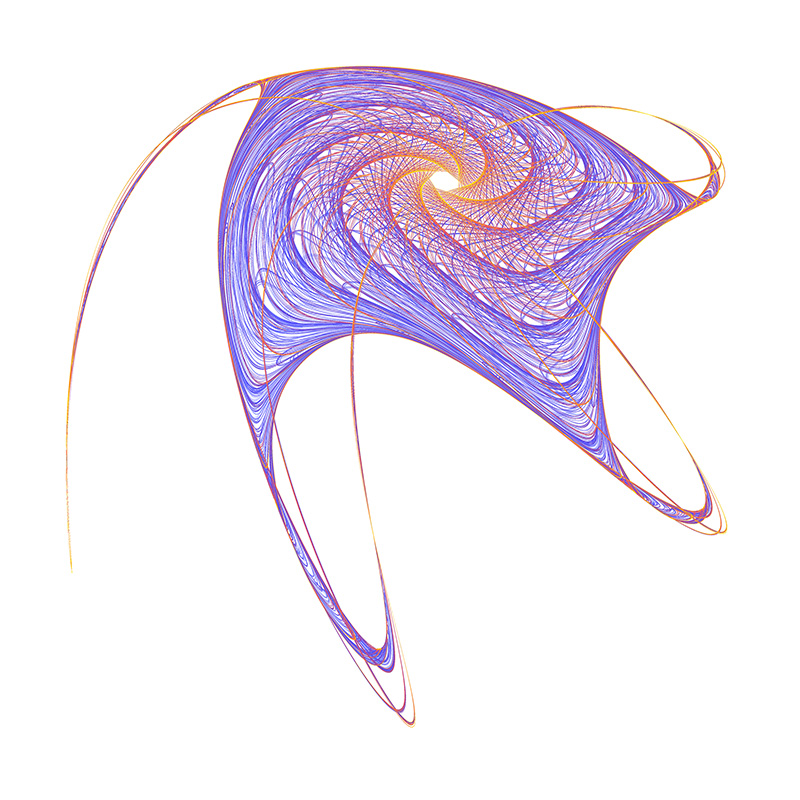GLXOESFTTPSV

What is this "GLXOESFTTPSV"? A nice innovation in the paper was to identify the attractors by their series a0... a11. This was done by choosing values for each between -1.2 to 1.2 in steps of 0.1 and mapping those to uppercase letter A through to Y. S, GLXOESFTTPSV represents a0 = -0.6, a1 = -0.1, a2 = 1.1, a3 = 0.2, a4 = -0.8, a5 = 0.6, a6 = -0.7, a7 = 0.7, a8 = 0.7, a9 = 0.3, a10 = 0.6, a11 = 0.9.MCRBIPOPHTBN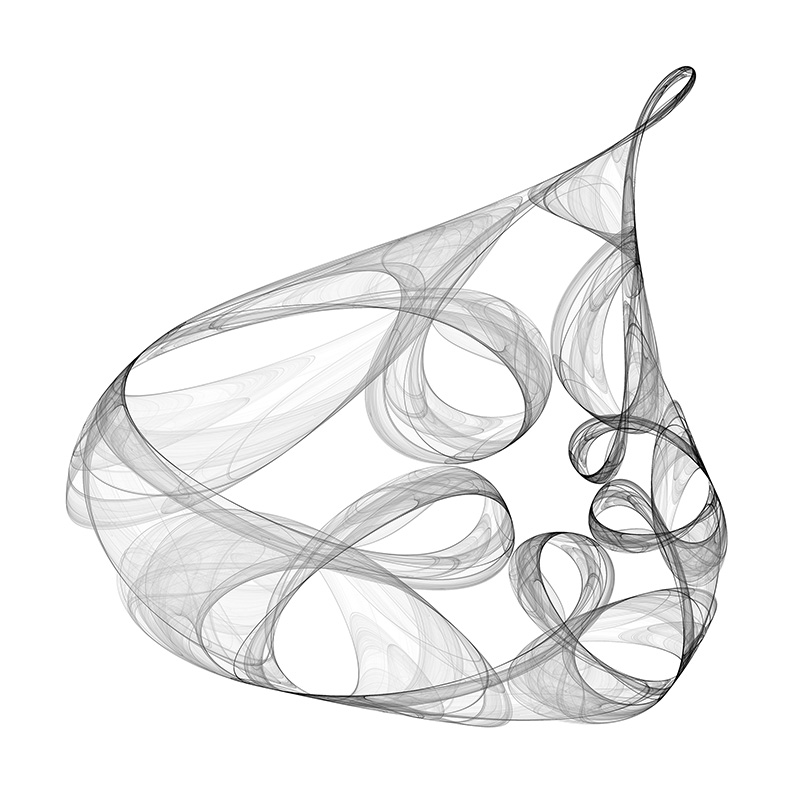VBWNBDELYHUL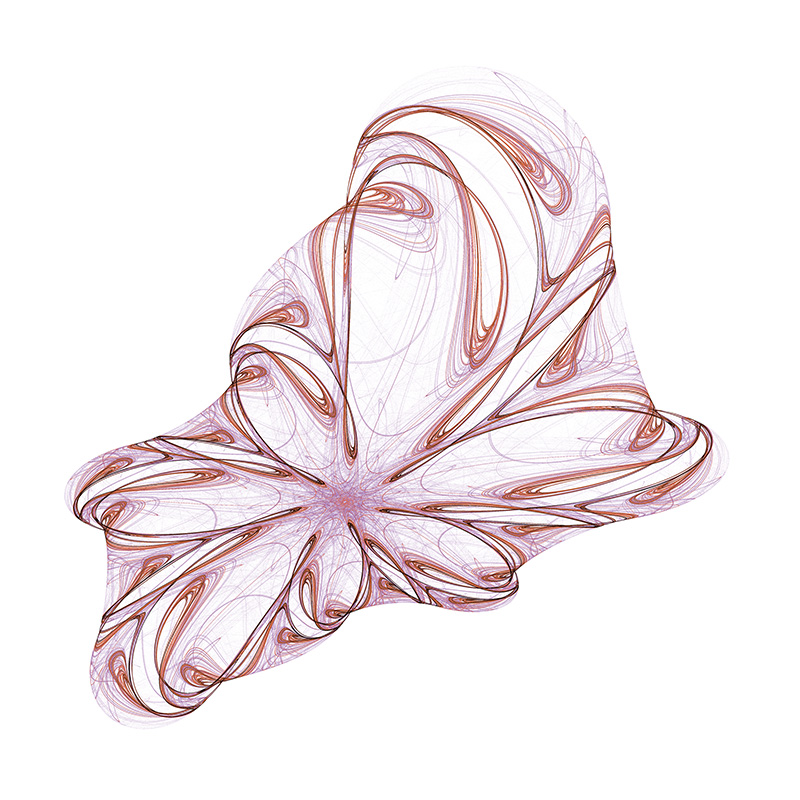FIRCDERRPVLDQFFVSLMJJCCRLUFBBFISGJYS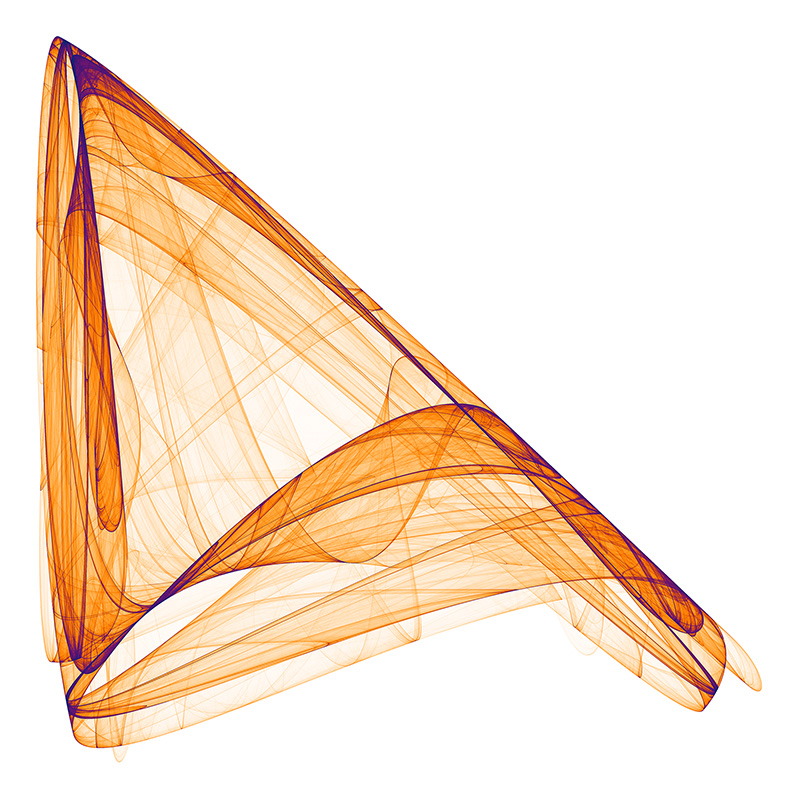EJYDREGLYQPV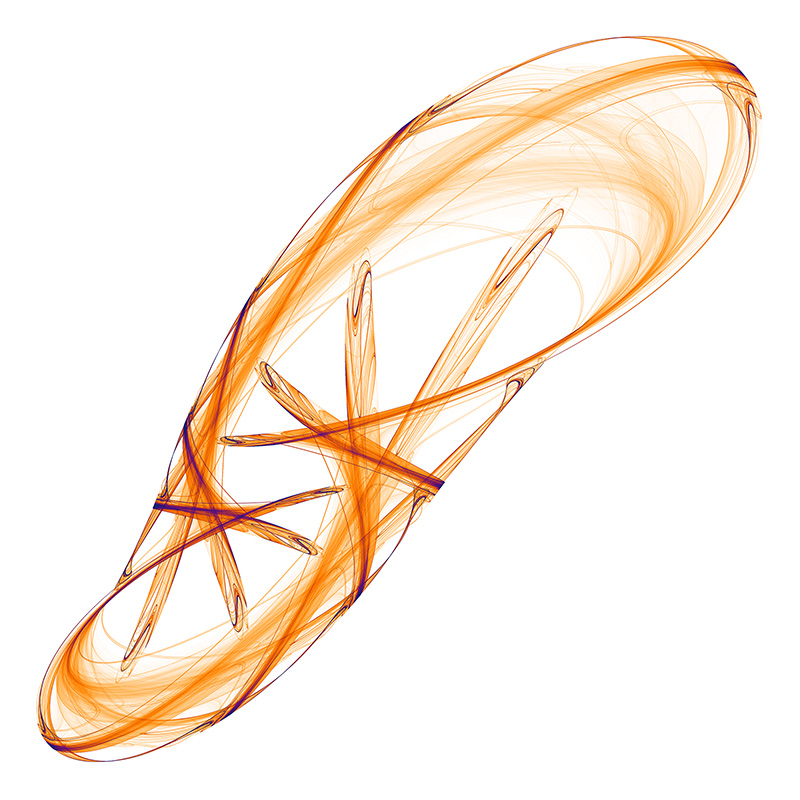HIYIWHOKNVCG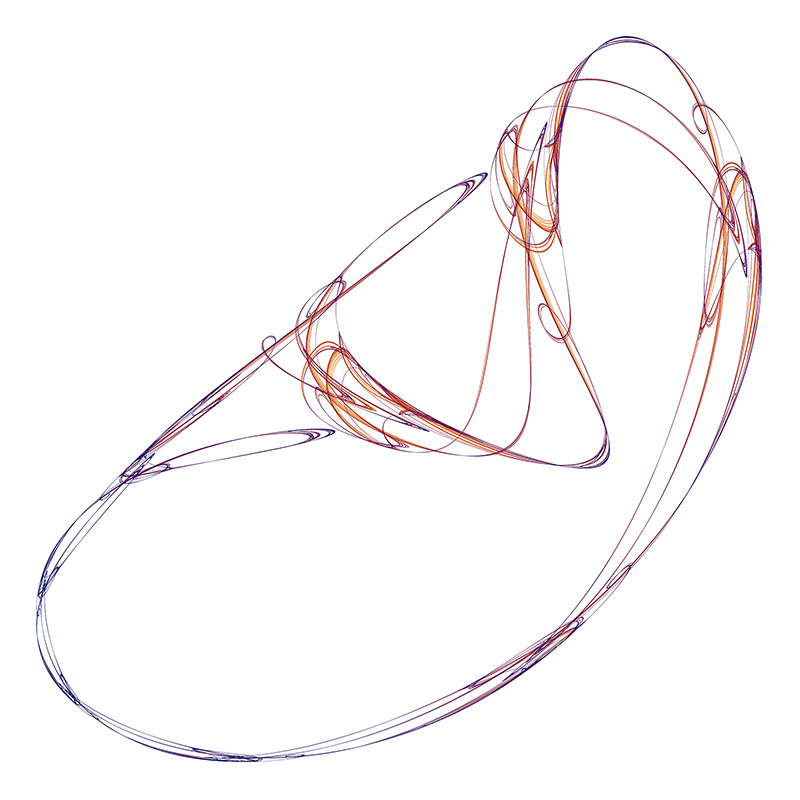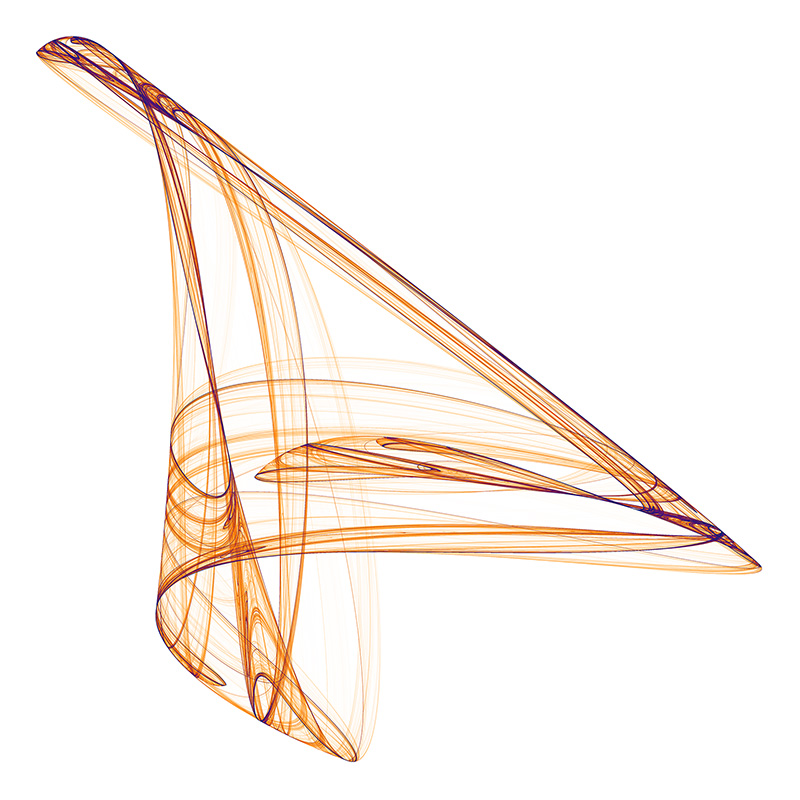OIHVGHAHGYRK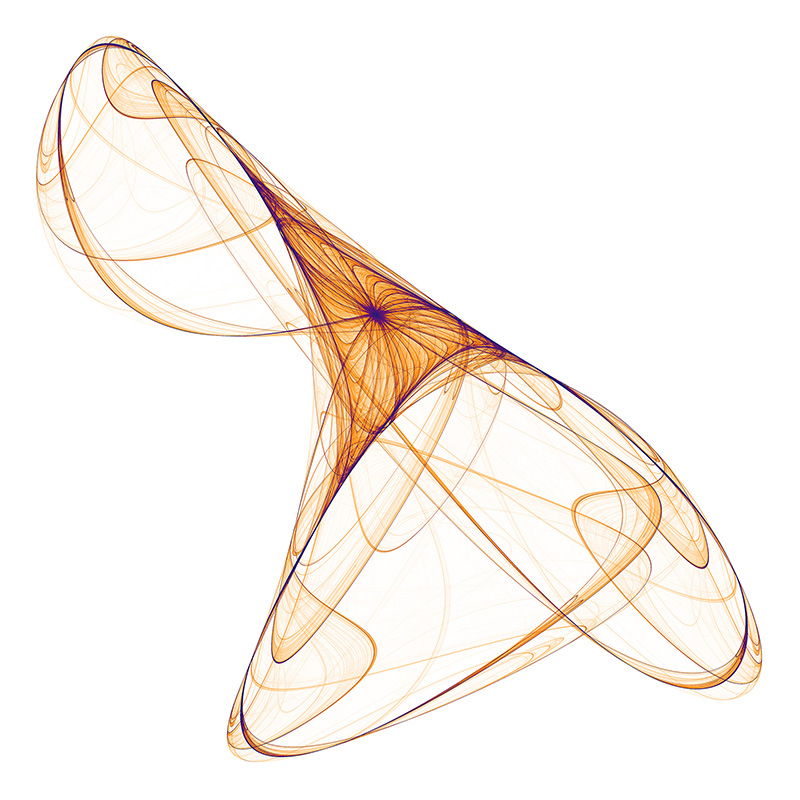RALLTIOBDULTWJJFXGXHTRPG

### Extension to 3D

The paper extends the notion to polynomials of 3 variables (x,y,z), this results in 30 parameters for a general 2 degree polynomial. This is written here as follows, noting the convention is different to that used in a subsquent republising of the paper.

xn+1 = a0 + a1xn + a2yn + a3zn + a4xn2 + a5yn2 + a6zn2 + a7xnyn + a8xnzn + a9ynzn
yn+1 = a10 + a11xn + a12yn + a13zn + a14xn2 + a15yn2 + a16zn2 + a17xnyn + a18xnzn + a19ynzn
zn+1 = a20 + a21xn + a22yn + a23zn + a24xn2 + a25yn2 + a26zn2 + a27xnyn + a28xnzn + a29ynzn

While the previous papers presented 2D projections onto a coordinate plane, here I populate a volume as a histogram and then render that volumetrically using ray casting. The volumes used in the examples here are 1000x1000x1000 voxels.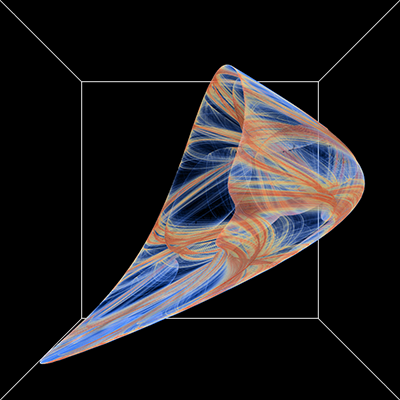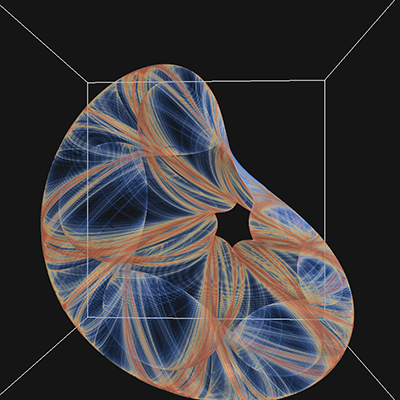JSSISBSCETFBRROIRRMRFQKPSMCJAA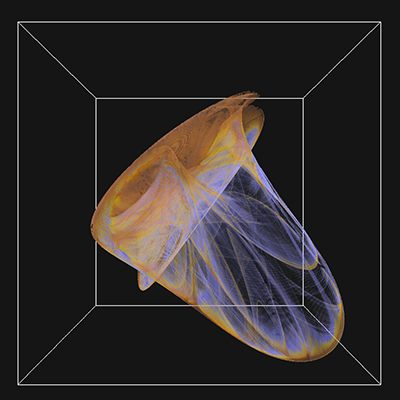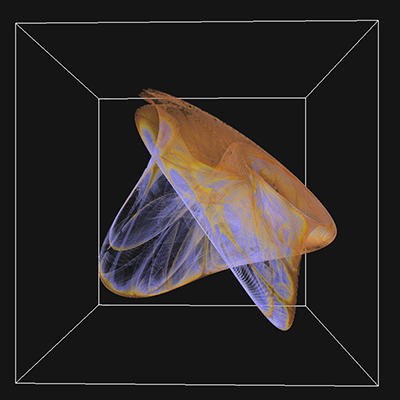MJTUNGHFURGOOQSJQFFNCJARKODAOB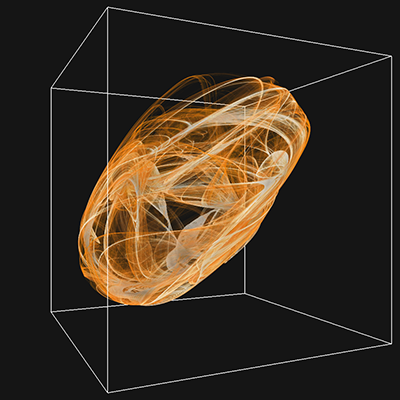AGUREBTODODUSKFUTGIFDDKOTJNSKF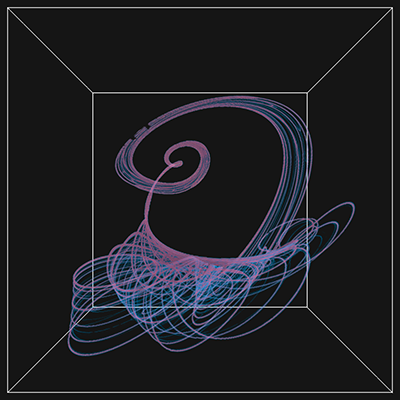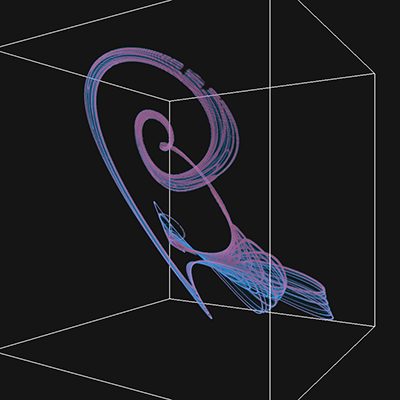OIDOMGJJBRSCUNJQBQOIBAGNDOEDFN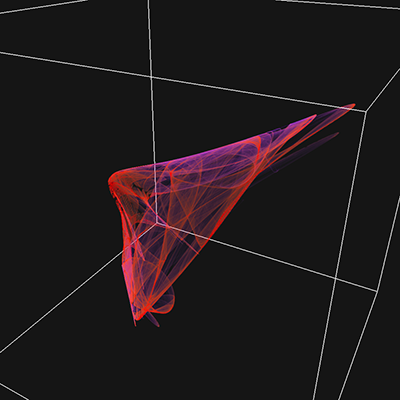GTAANKABNPCHROMCFCCSPUMUAHPTMN